#### You may also like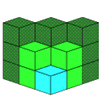If you had 36 cubes, what different cuboids could you make?### Construct-o-straws

Make a cube out of straws and have a go at this practical challenge.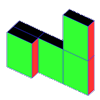### Cereal Packets

How can you put five cereal packets together to make different shapes if you must put them face-to-face?

# Making Cuboids

## Making Cuboids

Let's say you can only use two different lengths - $2$ units and $4$ units.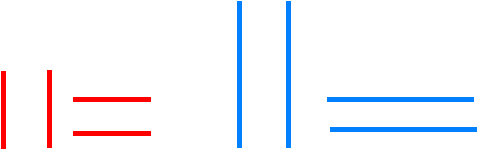If you are using these lengths to make the sides of rectangles, how many different ones can you make? (Squares are just special rectangles!)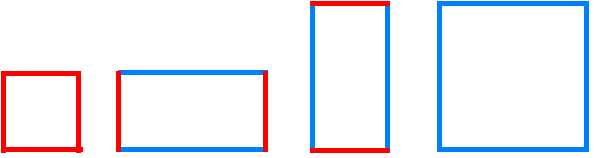There are three because two are the same - just rotated.

But we are not making $2$-dimensional rectangles!

We are going to make $3$-dimensional cuboids.

Using just these two lengths as the edges of the cuboids how many different ones can you make?

Here are two of them. How many more are there?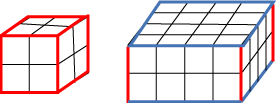It is a good idea to make them from squared paper and sticky tape, but you also need to find a way to record your results.

This is one way to show the results above, making a list.

 The smallest is $2 \times 2 \times2$ The middle sized one is $2 \times4 \times4$

You should find a way to record your results that makes sense to you.

How can you make sure that your cuboids are not the same if rotated?

How many different cuboids did you get?

But what if we have three different lengths: $2$ units, $3$ units and $4$ units?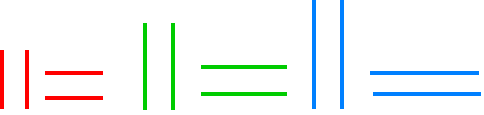How many different cuboids can you make now?

Make them from squared paper and sticky tape.

Find a way of showing what they look like.

Once they are made perhaps you can arrange them in order of size - but how will you work this out?

### Why do this problem?

This problem is one that concentrates on the length of the edges of cuboids rather than on the faces or vertices. It can help children to understand the nets of 3D shapes. It is a good precursor to lessons on finding the volume of cuboids.

### Possible approach

You could introduce this investigation with the 2D rectangles as is done in the text of the problem. Alternatively, you could start by showing the group two cuboids that you have made up beforehand. Have the nets available so the children can see how they are folded into the cuboid. This sheet of two nets might be useful. (You will need two copies to show both the nets and the made-up cuboids.)

When you have discussed the problem and what needs to be done children could work in pairs so that they are able to talk through their ideas with a partner. Supply $1$ cm$^2$ paper, scissors and sticky tape for making the models.

When introducing the third length, point out that the work done beforehand has not been wasted - the cuboids already made are just part of a now enlarged family. Some children may notice this for themselves.

Making a list or table is an important and useful way of checking that all possibilities have been included. This could be done at the end of the lesson. Show the group how to do this methodically so that none are included twice, such as $3 \times4 \times2$ and $2 \times4 \times3$. One way to do this is to list in order of the size of the numbers so that the above example will always be recorded as $2 \times3 \times4$.

Later, carefully constructed model cuboids along with their nets could be made for display.

The cuboids can also be sketched on isometric dotty paper.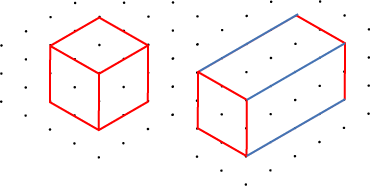### Key questions

Which would be a good cuboid to start on?
Where do the two rectangles that are the same size go on the net of the cuboid?

### Possible extension

Learners could investigate what how many cuboids will be possible when another length is added. Can they predict what would happen for five lengths?
$1$ length - $1$ cuboid
$2$ lengths - $4$ cuboids
$3$ lengths - $?$ cuboids
$4$ lengths - $?$ cuboids

### Possible support

Some children might find it easier to construct cuboids out of drinking straws and plasticine, or special construction kits.